Vector Functions And Space Curves

(requires JavaScript)

1. Find the domain of the vector function $\mathbf{r}\left(t\right)=⟨{t}^{2},\sqrt{t-1},\sqrt{5-t}⟩$.
$\left[1,5\right]$
2. Find $\underset{t\to {0}^{}}{\mathrm{lim}}⟨\mathrm{arctan}\left(t\right),{e}^{-2t},\frac{\mathrm{ln}\left(t\right)}{t}⟩$
The limit does not exist because $\frac{\mathrm{ln}\left(t\right)}{t}$ diverges to infinity as $t$ tends to $0$ from above.
3. Sketch the curve given by equation $\mathbf{r}\left(t\right)=⟨\mathrm{sin}\left(t\right),t⟩$ and indicate the direction in which $t$ increases.
4. Sketch the curve given by equation $\mathbf{r}\left(t\right)=⟨1,\mathrm{cos}\left(t\right),2\mathrm{sin}\left(t\right)⟩$ and indicate the direction in which $t$ increases.
5. Sketch the curve given by equation $\mathbf{r}\left(t\right)={t}^{2}\mathbf{i}+t\mathbf{j}+2\mathbf{k}$ and indicate the direction in which $t$ increases.
6. Find a vector equation and parametric equations for the line segment joining $P\left(1,0,1\right)$ and $Q\left(2,3,1\right)$.
Vector equation: $\mathbf{r}\left(t\right)=⟨2,3,1⟩-⟨1,3,0⟩t$.
Parametric equations: $x=2-t$, $y=3-3t$, $z=1$, where $0\le t\le 1$.
7. Match parametric equations with the given graphs. Explain.
1. $x=\mathrm{cos}\left(4t\right),\phantom{\rule{0.5em}{0ex}}y=t,\phantom{\rule{0.5em}{0ex}}z=\mathrm{sin}\left(4t\right)$.
2. $x=t,\phantom{\rule{0.5em}{0ex}}y={t}^{2},\phantom{\rule{0.5em}{0ex}}z={e}^{-t}$.
3. $x=t,\phantom{\rule{0.5em}{0ex}}y=\frac{1}{1+{t}^{2}},\phantom{\rule{0.5em}{0ex}}z={t}^{2}$.
4. $x={e}^{-t}\mathrm{cos}\left(10t\right),\phantom{\rule{0.5em}{0ex}}y={e}^{-t}\mathrm{sin}\left(10t\right),\phantom{\rule{0.5em}{0ex}}z={e}^{-t}$.
5. $x=\mathrm{cos}\left(t\right),\phantom{\rule{0.5em}{0ex}}y=\mathrm{sin}\left(t\right),\phantom{\rule{0.5em}{0ex}}z=\mathrm{sin}\left(5t\right)$.
6. $x=\mathrm{cos}\left(t\right),\phantom{\rule{0.5em}{0ex}}y=\mathrm{sin}\left(t\right),\phantom{\rule{0.5em}{0ex}}z=\mathrm{ln}\left(t\right)$.

I.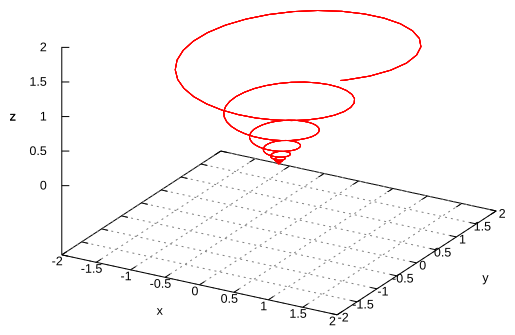II.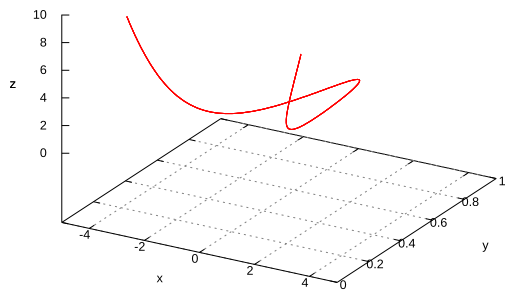III.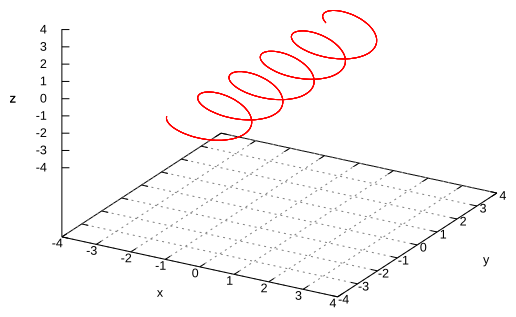IV.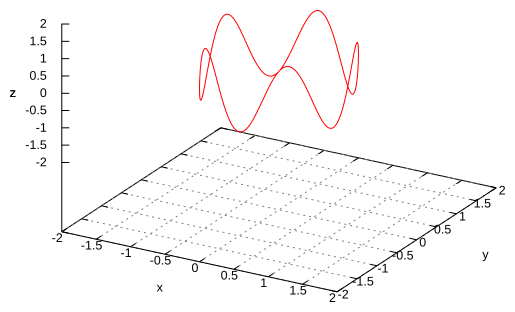V.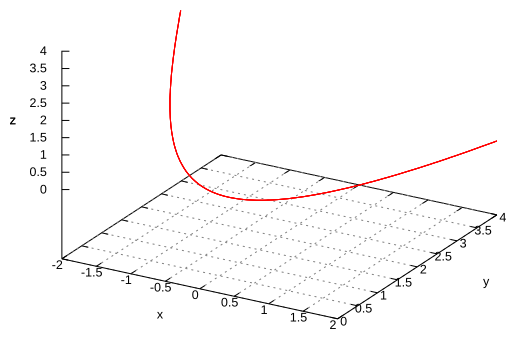VI.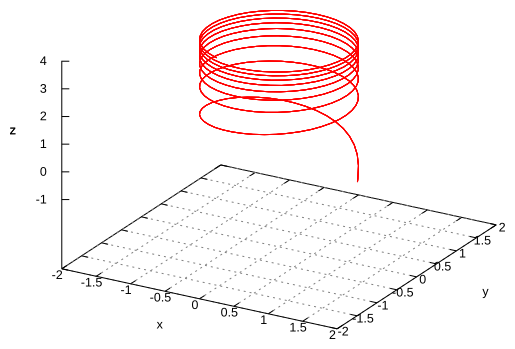III, V, II, I, IV, VI.
8. Use a computer to graph the curve given by the equation $\mathbf{r}\left(t\right)=⟨\mathrm{sin}\left(t\right),\mathrm{sin}\left(2t\right),\mathrm{sin}\left(3t\right)⟩$.
9. Two neutrons travel along the space curves ${\mathbf{r}}_{1}\left(t\right)=⟨t,{t}^{2},{t}^{3}⟩$ and ${\mathbf{r}}_{2}\left(t\right)=⟨1+2t,1+6t,1+14t⟩$. Will they collide? Do their paths intersect?
No collision; intersections at $⟨1,1,1⟩$ and $⟨2,4,8⟩$.